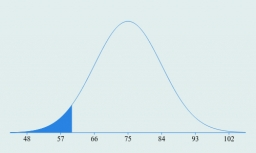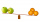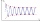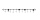# Find the mean

Find the number between 13 and 29.

m =  21

### Step-by-step explanation:

$m=\frac{13+29}{2}=21$Did you find an error or inaccuracy? Feel free to write us. Thank you!Tips to related online calculators
Looking for help with calculating arithmetic mean?
Looking for a statistical calculator?

#### You need to know the following knowledge to solve this word math problem:

We encourage you to watch this tutorial video on this math problem:

## Related math problems and questions:

• NumbersDetermine the number of all positive integers less than 4183444 if each is divisible by 29, 7, 17. What is its sum?
• Find the 24Find the difference between 2/7 and 1/21
• Find unknown numberWhat is the number between 50 and 55 that is divisible by 2,3,6,9?
• Seven timesWhich number seven times is just as higher as 27, how much is smaller than 29?
• Arithmetic averageThe arithmetic mean of the five numbers is exactly 8. The sum of these four numbers is 30. What is the fifth number?
• Closest natural numberFind the closest natural number to 4.456 to 44.56 and 445.6.
• Sort fractionsWhich of the following fractions is the largest? 29/36 5/6 7/9 3/4
• SixteenSixteen minus the quotient of 12 and what number is thirteen?
• RegroupingSubtract mixed number with regrouping: 11 17/20- 6 19/20
• Sum of four numbersThe sum of four consecutive natural numbers is 114. Find them.
• Digit sumFind the smallest natural number n, the digit sum of which is equal to 37.
• The sum 12The sum of 3 mixed numbers is 20 13/15. two of the numbers are 6 1/3 and 7 5/6. what is the third number?
• Divisible by nineHow many three-digit natural numbers in total are divisible without a remainder by the number 9?
• Between two mixedWhat is the rational number between 2 1/4 and 2 4/5?
• Result and remainderAfter dividing the unknown number by the number 23, the quotient 11 and the remainder 4 are formed. Find an unknown number.
• Four multiplesFour multiples of 6 he writes the following 12  24  56  72, which is correct?
• Evaluate 12Evaluate and simplify 9 divided by 7/6.